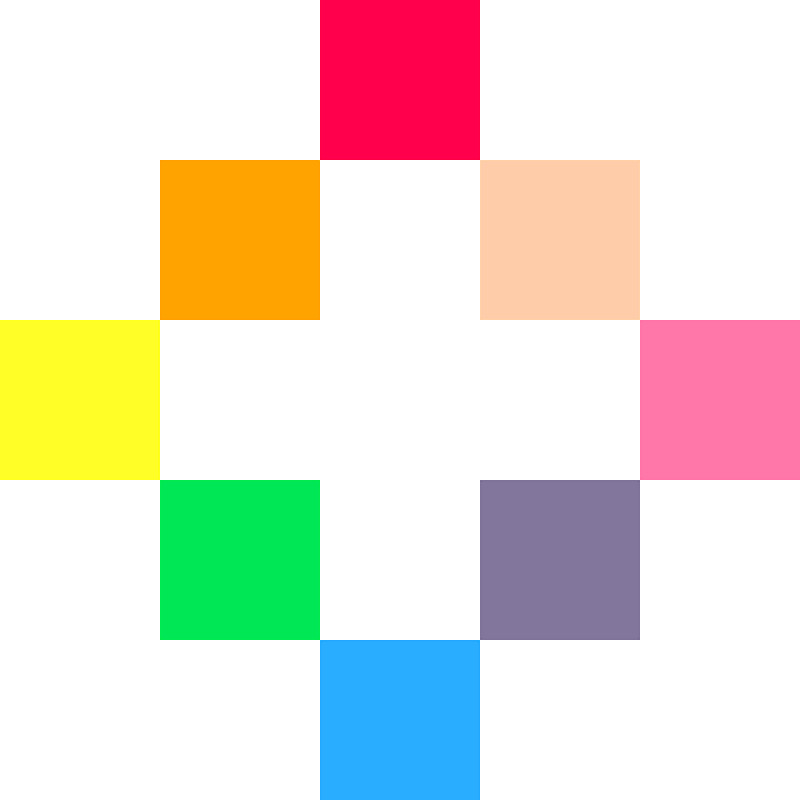## PICO-8Follow along with the video to learn how to control game states for a main menu!

The video moves quickly so pause whenever you need.

Make sure you understand why this code works or you won't be able to make your own game states later!

After you made this main menu, change it up! Try some of the challenges below to spark some ideas!

### Challenges!

After following the lesson, take on some of these challenges to test your knowledge and skills!

3. Add other options, like "Select a Character".

4. Add another Game State before starting the actual game, such as an Options Menu.

# Intro to Making a Main Menu!

## Game Sprites!What is a sprite?

• ## 1. Set up the Variables

What is a variable?
``````function _init()
end
``````

By setting up your variables inside of the `_init()` function, you can easily reset your game by calling `_init()`, and the variables here have access to any custom functions you write. That might come in handy later so it's a good practice.

``````--variables
x=63
y=63``````

The first variable is named `scene`. Then the equals sign tells the game to remember it with whatever we write next. `scene` holds a word (technically called a "string") and that string is the name of the scene (technically called a "game state") that we want to run.

The next variables are named `x` and `y`. Together these tell the game where the player character is positioned on the screen.

`x=63` and `y=63` because the screen has 128 pixels from left-to-right and top-to-bottom (# 0-127), so the screen center is at (63,63)

These are used to draw the player character in the center of the screen at the beginning of the game.• ## 2. Simple State Machine

``````--main update and draw

function _update()
elseif scene=="game" then
update_game()
end
end

function _draw()
elseif scene=="game" then
draw_game()
end
end``````

`_update()` makes changes to the game 30 times every second.

`_draw()` runs after _update and draws to the game screen 30 times every second.

`if scene=="menu" then` checks the "scene" variable for which state should be active.

You can do this for each game state that you want to create. Just add an `elseif` to the check for the other game states.

`update_menu()`

`draw_menu()`

`update_game()`

`draw_game()`

These are going to be our custom functions that become what `_update()` and `_draw()` will run, that are specific to the game state, when it is active.• ## 3. Specific Update Functions

``````--updates

if btnp(❎) then
scene="game"
end
end

function update_game()
if btn(⬅️) then x-=1 end
if btn(➡️) then x+=1 end
if btn(⬆️) then y-=1 end
if btn(⬇️) then y+=1 end

if btnp(❎) then
end
end``````

`function update_menu()` creates a custom function for the specific updating of the Main Menu scene.

`if btnp(❎) then` checks if the player pressed the X button and ignores if the player holds it down too long.

`scene="game"` changes the "scene" variable so that the game state will change to the actual game scene.`function update_game()` creates a custom function for the specific updating of the Game scene.

`if btn(⬅️) then x-=1 end ...` is the Simple Movement code.

Then we use the same check as in `update_menu()` function for switching the game state back to the Main Menu, only for demonstration purposes.

You would probably want to have a Game Over scene or a Win scene after your Game scene, and not just from a click of a button.• ## 4. Specific Draw Functions

``````--draws

cls()
print("press ❎ to start",30,63)
end

function draw_game()
cls()
spr(1,x,y)
end``````

`function draw_menu()` creates a custom function for the specific drawing of the Main Menu scene.

`cls()` clears the screen.

`print("press ❎ to start",30,63)` writes the words "press X to start" at position (30,63) which is about center.`function draw_game()` creates a custom function for the specific drawing of the Game scene.

`cls()` clears the screen.

`spr(1,x,y)` simply draws the sprite #1 at the coordinates (x,y) which change in the `update_game()` function based on the player's button presses.• ## Full Code!

``````--initialize
function _init()
--variables
x=63
y=63
end

-->8
--main update and draw

function _update()
elseif scene=="game" then
update_game()
end
end

function _draw()
elseif scene=="game" then
draw_game()
end
end

-->8

if btnp(❎) then
scene="game"
end
end

function update_game()
if btn(⬅️) then x-=1 end
if btn(➡️) then x+=1 end
if btn(⬆️) then y-=1 end
if btn(⬇️) then y+=1 end

if btnp(❎) then
end
end

-->8
--draws

cls()
print("press ❎ to start",30,63)
end

function draw_game()
cls()
spr(1,x,y)
end
``````

`-->8` is a new tab in Pico-8. They don't appear inside the Pico-8 code editor.

•`font`